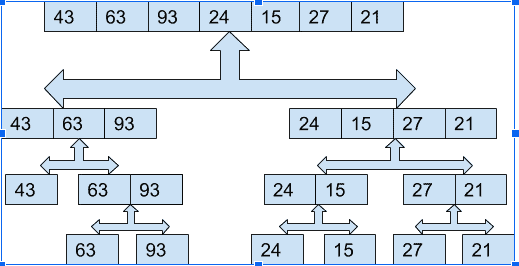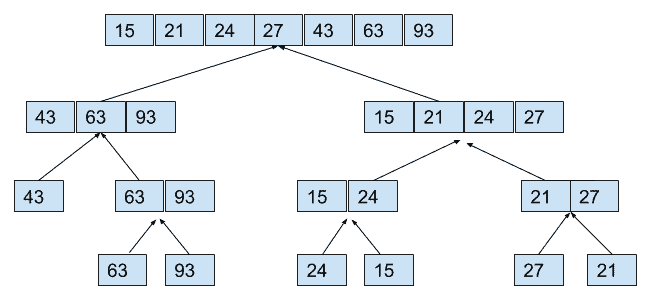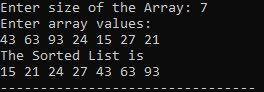# Merge Sort in C++: The Complete Guide

Merge sort in C++ first divides an array into equal halves and then combines them in a sorted manner. The Merge sort is an efficient and comparison-based algorithm to sort an array or a list of integers.

Merge Sort keeps dividing the list into equal halves until it can no more be divided. By definition, it is sorted if there is only one element in the list. Then, Merge Sort combines the smaller sorted lists keeping the new list sorted.

The divide and concur have the following three tasks.

1. Divide the problems into subproblems similar to the original but smaller in size.
2. Conquer the sub-problems recursively. If they are smaller in size, straightforwardly solve them.
3. Combine those solutions into a solution, the answer to the original problem.

Let’s understand the merge sort in C++ and then write a program demonstrating it.

### How divide and conquer Algorithm works in Merge Sort

Sorting Problem: Sort a sequence of n elements into non-decreasing order.

Divide:  Divide the n-element sequence to be sorted into two subsequences of n/2 elements each

Conquer:  Sort the two subsequences recursively using merge sort.

Combine:  Merge the two sorted subsequences to produce the sorted answer.

The top-down merge sort approach is a methodology that uses the recursion mechanism. It starts from the Top and proceeds downwards, with each recursive turn asking the same question, such as “What is required to be done to sort the array?” and having an answer as, “split an array into two, make a recursive call, and merge the results.” until one gets to the bottom of an array tree.

### Example

Okay, before going to the code, first understand a model of Merge Sort; this will help you know the code.

Let us consider a list of integers:

 43 63 93 24 15 27 21

So, as per the algorithm, we first need to divide the list length (n) into two parts until they are divided into one node.

So here, n=7 now n/2=3. Thus, the first part will be 3 integers, and the second part will be of 7-3=4 part.

Note: If the list size is even, both sides will be divided equally. But for the case of ODD smaller size will be on the left side, and the more significant will be on the right side.

So, after Step 1, the list will be divided like the following.

 43 63 93 24 15 27 21

Like this again, we will divide each of the lists by 2. The left side list will be in  1+2, and the right side will appear in the 2+2 part. The same thing will happen again until they all get separated. So, here we will see the final list after the divide method. See the following figure.Okay, after all the Divide operations are performed, we will perform Conquer and Sort the two subsequences recursively using merge Sort and Combine, that is, Merge the two sorted subsequences to produce the sorted answer operation.

So here, we will see what will be the result after each step:So, what happened here is… First, it compared two sub-sequences; for example, 63 and 93 are checked and placed in ascending order after the merge. Similarly, 27 and 21 are checked and then placed ascending after merging.

Again in step two, 43,63,93 were checked and placed in ascending order after merge. In this way, we got our final shortlist.

 15 21 24 27 43 63 93

## Pseudo Code for Merge Sort

Merge Sort Pseudo Code is the following.

```procedure mergesort( var a as array )
if ( n == 1 ) return a

var l1 as array = a ... a[n/2]
var l2 as array = a[n/2+1] ... a[n]

l1 = mergesort( l1 )
l2 = mergesort( l2 )

return merge( l1, l2 )
end procedure

procedure merge( var a as array, var b as array )

var c as array
while ( a and b have elements )
if ( a > b )
add b to the end of c
remove b from b
else
add a to the end of c
remove a from a
end if
end while

while ( a has elements )
add a to the end of c
remove a from a
end while

while ( b has elements )
add b to the end of c
remove b from b
end while

return c

end procedure```

## C++ Merge Sort Program

See the following mergesort.cpp file.

```#include<bits/stdc++.h>
using namespace std;
int Merge(int A[],int p, int q,int r)
{

int n1,n2,i,j,k;
//size of left array=n1
//size of right array=n2
n1=q-p+1;
n2=r-q;
int L[n1],R[n2];
//initializing the value of Left part to L[]
for(i=0;i<n1;i++)
{
L[i]=A[p+i];
}
//initializing the value of Right Part to R[]
for(j=0;j<n2;j++)
{
R[j]=A[q+j+1];
}
i=0,j=0;
//Comparing and merging them
//into new array in sorted order
for(k=p;i<n1&&j<n2;k++)
{
if(L[i]<R[j])
{
A[k]=L[i++];
}
else
{
A[k]=R[j++];
}
}
//If Left Array L[] has more elements than Right Array R[]
//then it will put all the
// reamining elements of L[] into A[]
while(i<n1)
{
A[k++]=L[i++];
}
//If Right Array R[] has more elements than Left Array L[]
//then it will put all the
// reamining elements of L[] into A[]
while(j<n2)
{
A[k++]=R[j++];
}
}
//This is Divide Part
//This part will Divide the array into
//Sub array and then will Merge them
//by calling Merge()
int MergeSort(int A[],int p,int r)
{
int q;
if(p<r)
{
q=(p+r)/2;
MergeSort(A,p,q);
MergeSort(A,q+1,r);
Merge(A,p,q,r);
}
}
int main()
{
int n;
cout<<"Enter size of the Array: ";
cin>>n;
int A[n],i;
cout<<"Enter array values:\n";
for(i=0;i<n;i++)
cin>>A[i];
//Calling the MergeSort()
//First we are passing the array
//the start index that is 0
//and the size of the array n

MergeSort(A,0,n-1);
cout<<"The Sorted List is\n";
for(i=0;i<n;i++)
{
cout<<A[i]<<" ";
}
return 0;
}```

The output is the following.## Time Complexity for Merge Sort

Running time T(n) of Merge Sort:

1. Divide: computing the middle takes Θ(1)
2. Conquer: solving 2 sub-problems takes 2T(n/2)
3. Combine: merging n elements takes Θ(n)
4. Total time complexity is the following.
```T(n) = Θ(1)
if n = 1T(n) = 2T(n/2) + Θ(n)
if n > 1
T(n)= 2 T(n/2) + n
= 2 ((n/2)log(n/2) + (n/2)) + n
= n (log(n/2)) + 2n
= n log n – n + 2n
= n log n + n
= O(n log n )```

The above recurrence can be solved using the Recurrence Tree or the Master method. However, it falls in case II of the Master Method, and the solution of the repetition is O(n log n).

The time complexity of the Merge Sort is O(n log n) in all 3 cases (worst, average, and best) as the merge sort divides the array into two halves and takes linear time to merge two halves.

It is one of the most respected algorithms, with the worst-case time complexity being Ο(n log n).

## Applications of Merge Sort

1. Merge Sort helps sort linked lists in O(nLogn) time.
2. It is used in Inversion Count Problem.
3. We can use it in External Sorting.

That’s it for this tutorial.

Categories C++

This site uses Akismet to reduce spam. Learn how your comment data is processed.